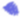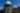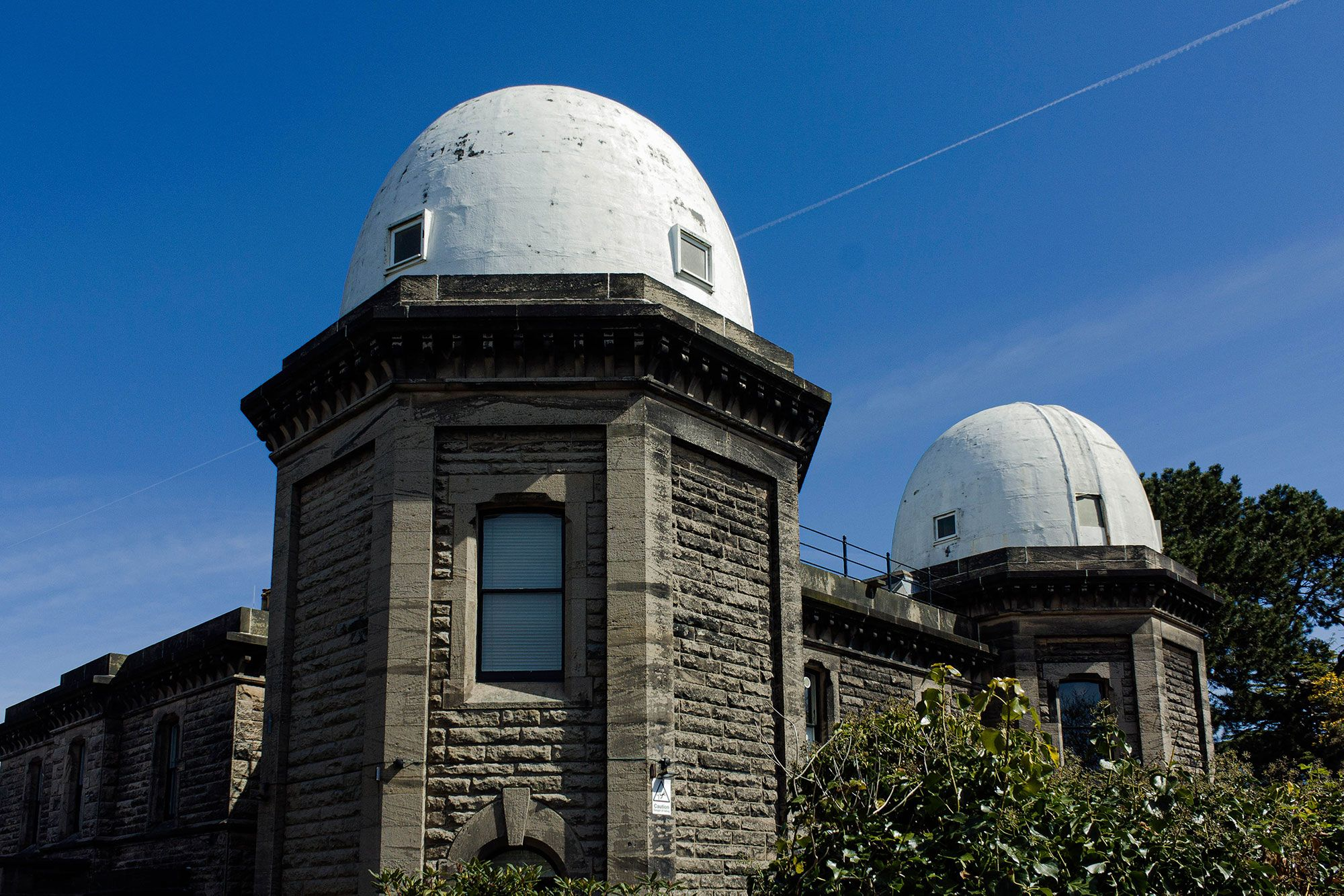## ((((((((((((((((((((((((((((((((((((((((((((((((((((((((((((((((((((((((((((((((((((((((((((((((((((((((((((((((((((((((((((((((((((((((((((((((((((((((((((((((((((((((((((((((((((((((((((((((((((((((The Project))))))))))))))))))))))))))))))))))))))))))))))))))))))))))))))))))))))))))))))))))))))))))))))))))))))))))))))))))))))))))))))))))))))))))))))))))))))))))))))))))))))))))))))))))))))))))))))))))))))))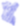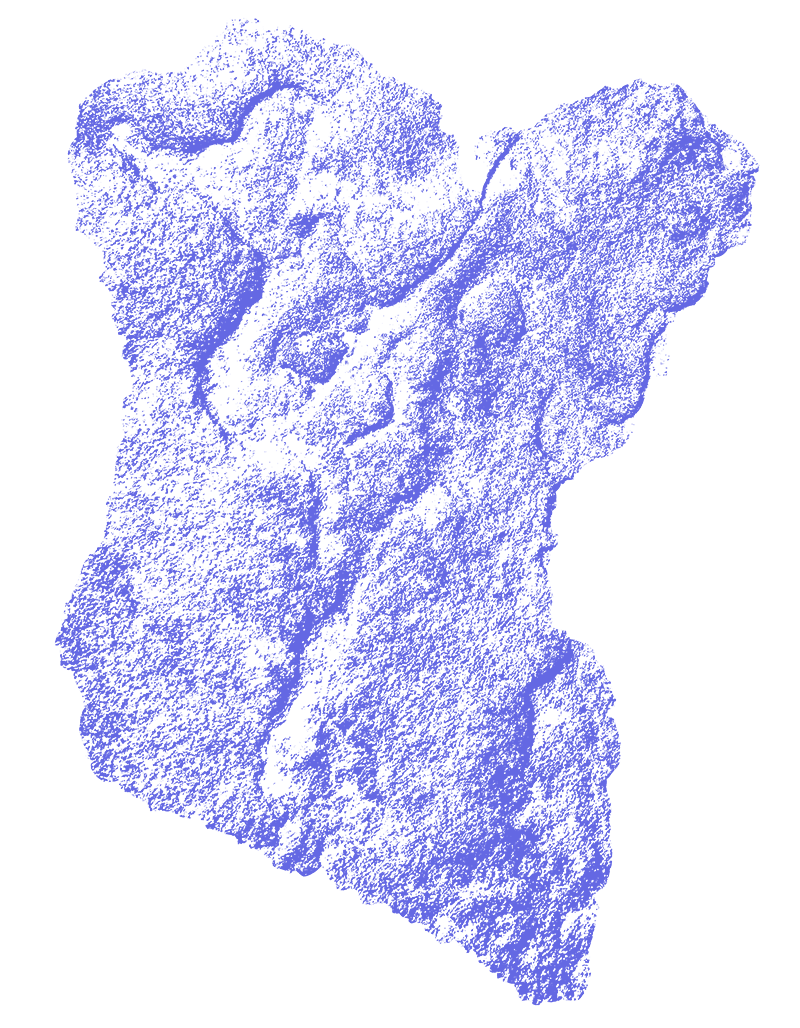Bidston Observatory Artistic Research Centre (BOARC) is a self-organising study site for research, communality and experimentation.

It is not for profit, and focuses on providing a low-cost, temporary work space for individuals and groups so they can to come together and take the time needed to develop their practices and projects. This can be in the form of nightly stays for up to a month, or day use of the building.

BOARC welcomes a wide diversity of fields, such as art, music and theatre, to philosophy, bodywork, social activism, environmental science, culture and technology.

Based in a historical landmark, it is a space where discussion can easily flow from the kitchen to the sound recording studio, and ideas can bounce between the domes and basement workspace.

Main kitchen and workspace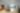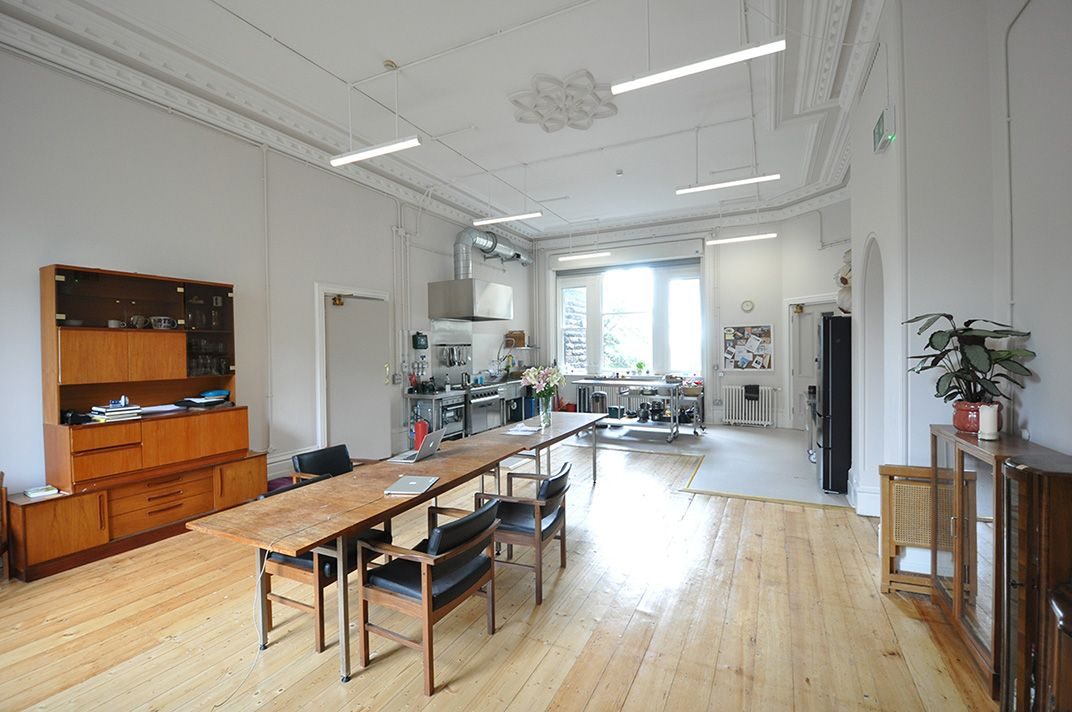There is no formal application to stay here – all are welcome to use this space. There is zero requirement to present your project and BOARC does not seek to lay claim to any work produced here. It is a flexible, non-pressured environment, where all types of practice are welcomed and valued.

Surrounded by beautiful woodland, it is just 30 minutes to Liverpool city centre.

Basement workspace, below main kitchen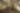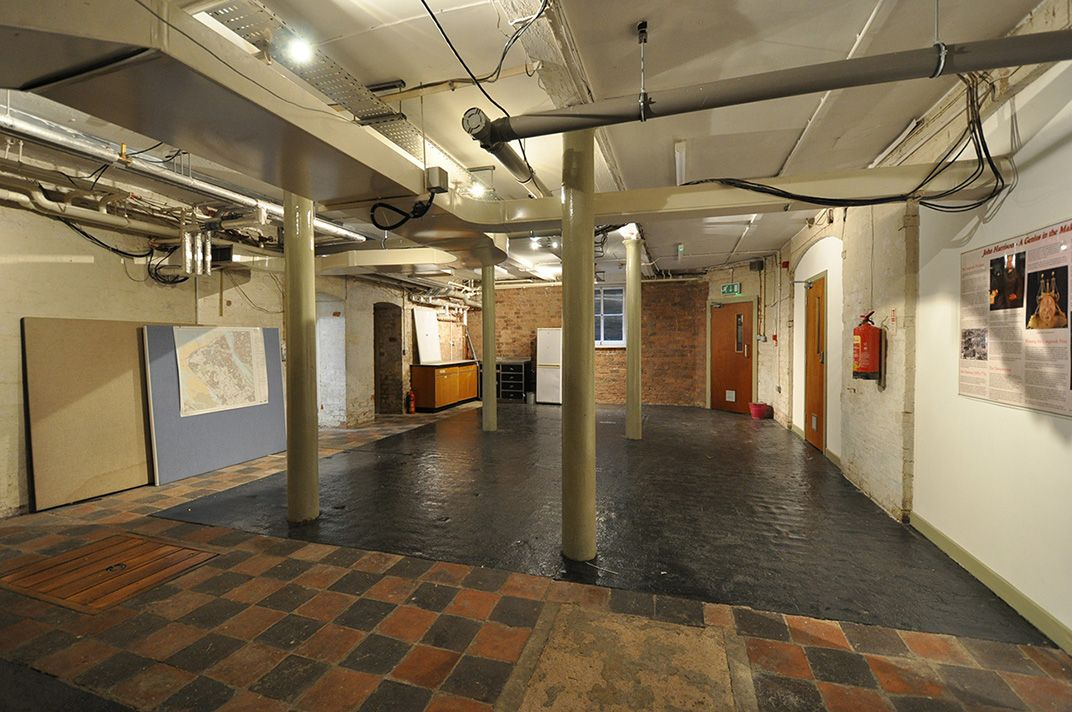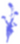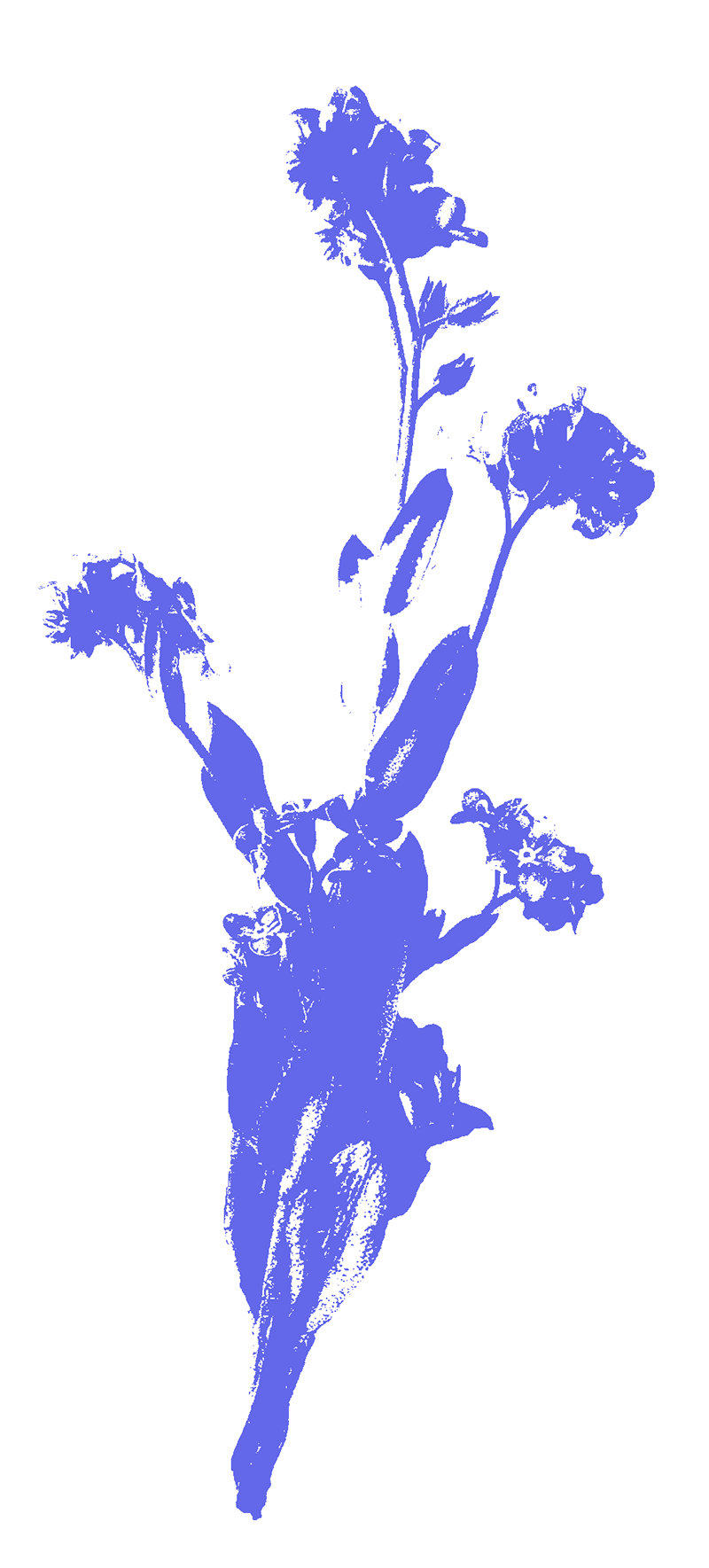## ((((((((((((((((((((((((((((((((((((((((((((((((((((((((((((((((((((((((((((((((((((((((((((((((((((((((((((((((((((((((((((((((((((((((((((((((((((((((((((((((((((((((((((((((((((((((((((((((((((((((Values and Accountability))))))))))))))))))))))))))))))))))))))))))))))))))))))))))))))))))))))))))))))))))))))))))))))))))))))))))))))))))))))))))))))))))))))))))))))))))))))))))))))))))))))))))))))))))))))))))))))))))))))))

Before booking, we ask that everyone read our 4 key values and accountability agreement. These will give you an idea if BOARC can support the needs of your practice.

Valuing experimentation

To support experimentation BOARC tries to move towards certain principals.

We think these make us slightly different to most residency sites, allowing for more radical ways of thinking. In short; No judgement, No pressure, No capture and No hierarchy of practice.

Supporting self-education and self-organisation

BOARC is a space that needs activating by others. We mean what we say, organising here is as simple as getting in touch to start a conversation.

Mutual trust and being in community

BOARC is an offer and a proposition. It offers a subsidised space outside the strains of daily life and proposes that these conditions support peoples natural impulse to also offer what they can.

Being accountable for ourselves

BOARC aims to be accessible and welcoming to all, but recognises that this is a collective responsibility. Our accountability agreement is an evolving practice and vital to how the space runs.

Efflorescence in the basement. Crystalline salt deposits forming in the basement work spaces as they dried out during renovations.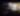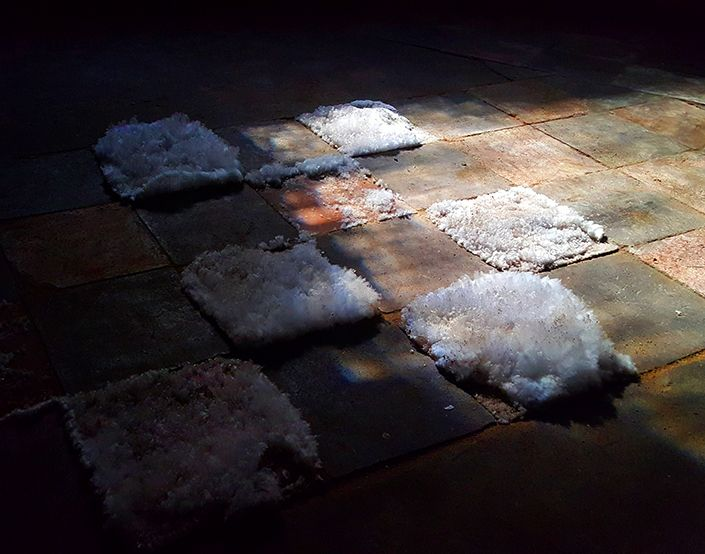Accountability Agreement

Please read thoroughly. Whilst it isn’t essential to agree with it exactly as it’s written, we ask that you consider it and make a decision for yourself whether you feel aligned.